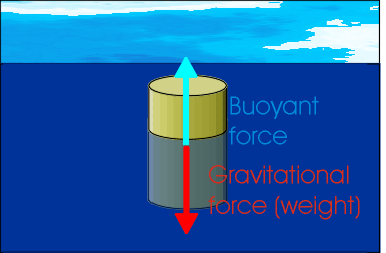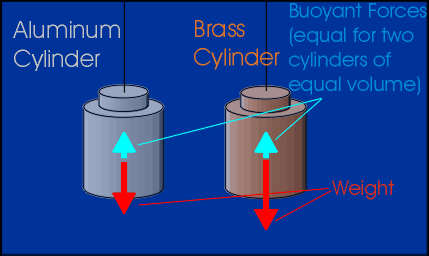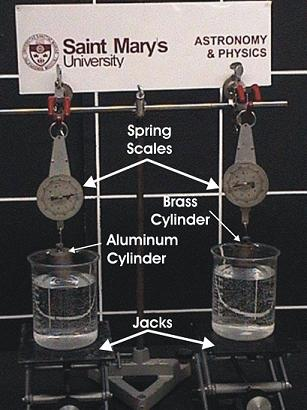### Two cylinders with different densities are lowered into water to show Archimedes' Principle.

WATCH THE VIDEO

Teachable Topics

• Buoyancy
• Density

Theory:

An object immersed in water is acted on by a buoyant force. This is a force caused by the pressure of the water on the object, and acts upwards against the force of gravity. Because this force opposes gravity, it can make certain objects float. Even objects that would otherwise sink appear ligher when they're held in water.

The famous Italian scientist, Archimedes (287 B.C - 212 B.C.), studied the phenomenon of objects appearing lighter when submerged in water. Archimedes' Principle states the following:

The buoyant force on an object which is submerged in a fluid is equal to the weight of the fluid displaced by the object.Figure 1: Forces acting on a floating object

As an example of this principle, imagine two cylinders that have different weights, but that have the same volume. When they are lowered into water, they will each displace the same volume. Archimedes' Principle therefore says that the buoyant force on the two cylinders will be the same. This means that the weights of the two cylinders should decrease by the same amount.Figure 2: Comparing floating objects of different mass & material

Apparatus:

• Two lab jacks
• One aluminum cylinder and one brass cylinder of the same volume
• A second aluminum cylinder with a different weight than the first
• Two beakers
• Water
• Clamps
• Two spring scales

Procedure:

1. Set up the apparatus as shown in the picture below, with the two weights hanging above the water's surface.
2. Elevate the two beakers sitting on the jacks at a constant speed. As the 130g aluminum cylinder, and 376g brass cylinder, displaces the water you will see a mass drop in the spring scales. The two masses are not the same weight or density. However they both possess equals volumes, and thus both displaces the same amount of water, and mass.
3. Lower the two beakers, and replace the 376g brass cylinder with the 90g aluminum cylinder. Repeat the same procedure as stated above. This time around the two cylinders have the same density but different masses and volumes. Once they have been displaced in the beakers of water, you will see the weight decrease in the 130g aluminum as before, and also in the aluminum cylinder too. Since the 90g aluminum has less volume it displaces less water.Figure 3: Apparatus